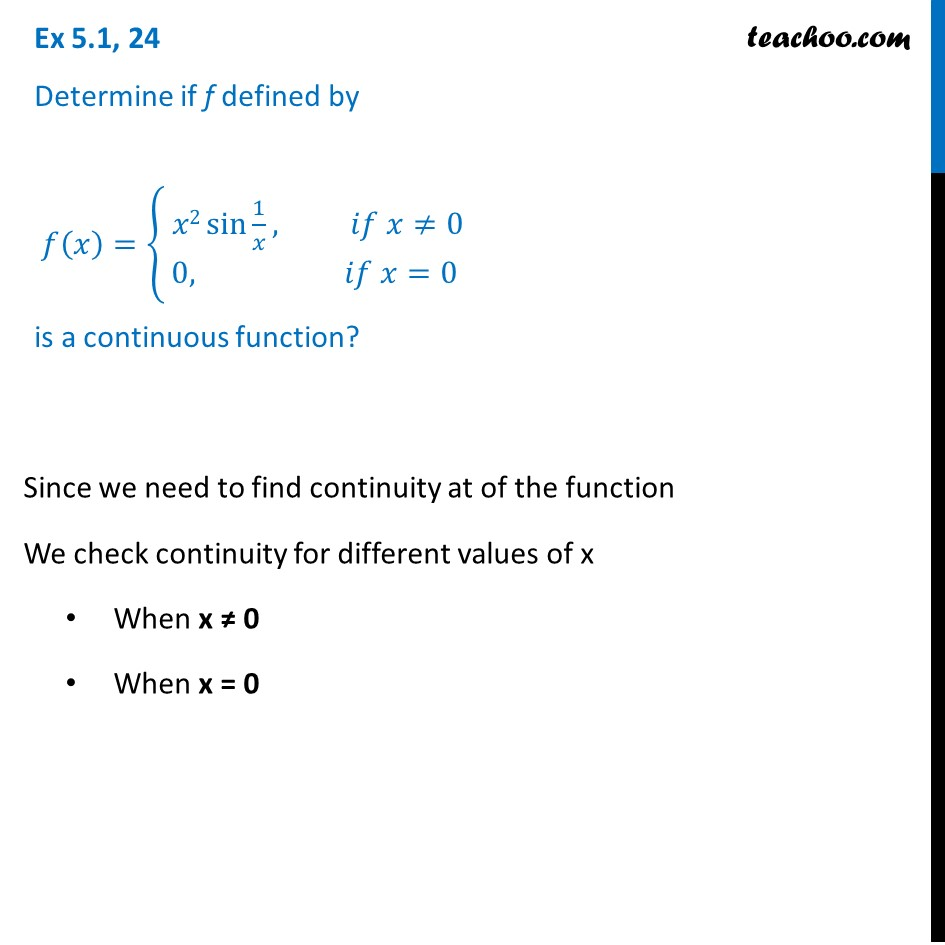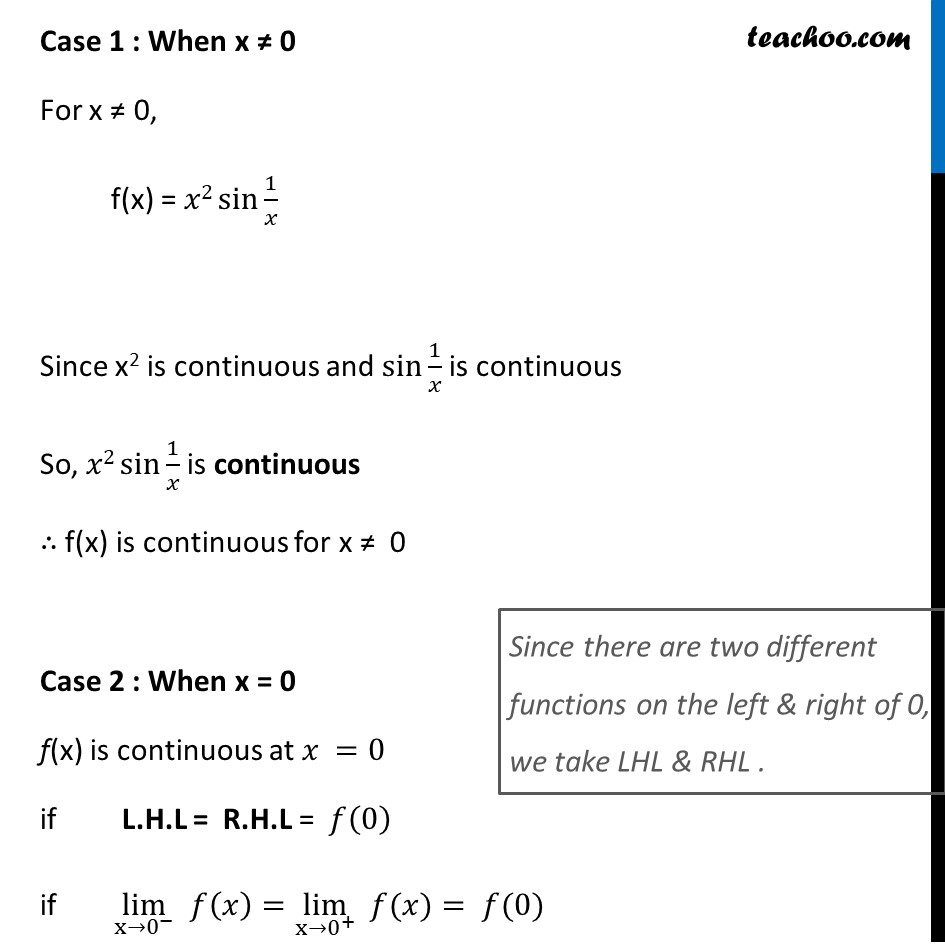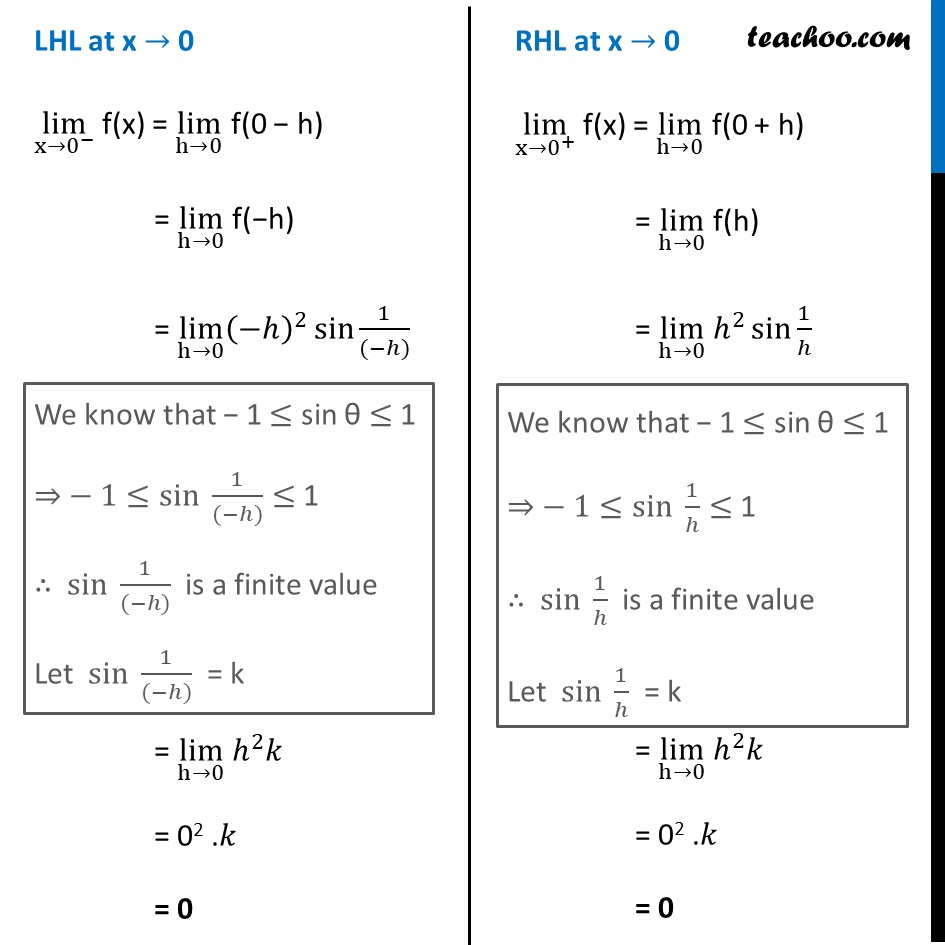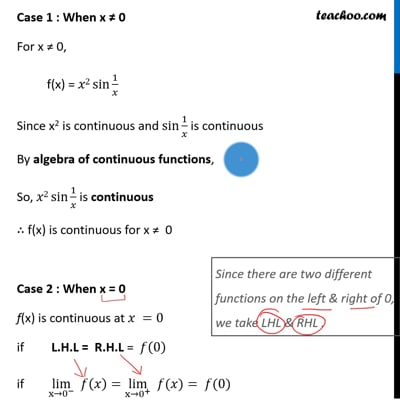Ex 5.1

Chapter 5 Class 12 Continuity and Differentiability
Serial order wiseThis video is only available for Teachoo black users

Introducing your new favourite teacher - Teachoo Black, at only ₹83 per month

### Transcript

Ex 5.1, 24 Determine if f defined by 𝑓(𝑥)={█( 𝑥2 sin⁡〖1/𝑥〗, 𝑖𝑓 𝑥≠0@&0, 𝑖𝑓 𝑥=0)┤ is a continuous function? Since we need to find continuity at of the function We check continuity for different values of x When x ≠ 0 When x = 0 Case 1 : When x ≠ 0 For x ≠ 0, f(x) = 𝑥2 sin⁡〖1/𝑥〗 Since x2 is continuous and sin⁡〖1/𝑥〗 is continuous So, 𝑥2 sin⁡〖1/𝑥〗 is continuous ∴ f(x) is continuous for x ≠ 0 Case 2 : When x = 0 f(x) is continuous at 𝑥 =0 if L.H.L = R.H.L = 𝑓(0) if lim┬(x→0^− ) 𝑓(𝑥)=lim┬(x→0^+ ) " " 𝑓(𝑥)= 𝑓(0) Since there are two different functions on the left & right of 0, we take LHL & RHL . LHL at x → 0 lim┬(x→0^− ) f(x) = lim┬(h→0) f(0 − h) = lim┬(h→0) f(−h) = lim┬(h→0) (−ℎ)^2 sin⁡〖1/((−ℎ))〗 = lim┬(h→0) ℎ^2 𝑘 = 02 .𝑘 = 0 We know that − 1 ≤ sin θ ≤ 1 ⇒ − 1≤〖sin 〗⁡〖1/((−ℎ))〗≤ 1 ∴ 〖sin 〗⁡〖1/((−ℎ))〗 is a finite value Let 〖sin 〗⁡〖1/((−ℎ))〗 = k RHL at x → 0 lim┬(x→0^+ ) f(x) = lim┬(h→0) f(0 + h) = lim┬(h→0) f(h) = lim┬(h→0) ℎ^2 sin⁡〖1/ℎ〗 = lim┬(h→0) ℎ^2 𝑘 = 02 .𝑘 = 0 We know that − 1 ≤ sin θ ≤ 1 ⇒ − 1≤〖sin 〗⁡〖1/ℎ〗≤ 1 ∴ 〖sin 〗⁡〖1/ℎ〗 is a finite value Let 〖sin 〗⁡〖1/ℎ〗 = k And, f(0) = 0 Hence, L.H.L = R.H.L = 𝑓(0) ∴ f is continuous at x=0 Hence, 𝒇(𝒙) is continuous for all real number